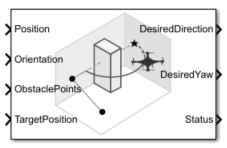# Obstacle Avoidance

Compute obstacle-free direction using range sensor data and target position

• Library:
• UAV Toolbox / Algorithms

•## Description

The Obstacle Avoidance block computes an obstacle-free direction using range sensor data and target position.

## Ports

### Input

expand all

Position of the UAV, specified as a vector of the form ```[x; y; z]```, in meters.

Example: `[1; 1; 1]`

Data Types: `double`

Orientation of the UAV, specified as a quaternion vector of the form ```[w; x; y; z]```.

Example: `[1; 0; 0; 0]`

Data Types: `double`

Positions of the obstacles, specified as an N-by-3 matrix with rows of the form ```[x y z]```, in meters. N is the number of obstacle points.

Example: `[1 1 1; 2 2 2]`

Data Types: `double`

Position of the target, specified as a vector of the form ```[x; y; z]```, in meters.

Example: `[2; 3; 4]`

Data Types: `double`

### Output

expand all

Desired direction, returned as a vector of the form ```[x; y; z]```, in meters.

Data Types: `double`

Desired yaw, returned as numeric scalar in radians in the range of ```[-pi, pi]```.

Data Types: `double`

Status of the obstacle-free direction, returned as `0`, `1`, `2`, or `3`.

• `0` — An obstacle-free direction is found.

• `1` — No obstacle-free direction is found.

• `2` — An obstacle-free direction is found but is close to the obstacle.

• `3` — No obstacle-free direction is found and is close to obstacle.

Data Types: `uint8`

## Parameters

expand all

Main

Specify the minimum and maximum limits of the range sensor as a vector of the form ```[min max]```, with values in meters.

Data Types: `double`

Specify the minimum and maximum horizontal field of view limits of the range sensor as a vector of the form ```[min max]```, with values in degrees.

Data Types: `double`

Specify the minimum and maximum vertical field of view limits of the range sensor as a vector of the form ```[min max]```, with values in degrees.

Data Types: `double`

Specify the mounting location of the sensor on the UAV as a vector of the form ```[x y z]```, with values in meters.

Data Types: `double`

Specify the orientation of the sensor mounted on the UAV as a vector of the form ```[roll pitch yaw]```, with values in degrees.

Data Types: `double`

This dimension defines the smallest circle that can circumscribe your vehicle, in meters. The vehicle radius is used to account for vehicle size when computing the obstacle-free direction.

Data Types: `double`

The safety distance specifies, in meters, the space accounted for between the UAV and obstacles in addition to the vehicle radius. The vehicle radius and safety distance are used to compute the obstacle-free direction.

Data Types: `double`

Specify whether to simulate the model using `Interpreted execution` or `Code generation`.

• `Interpreted execution` — Simulate the model using the MATLAB® interpreter. This option reduces startup time, but has a slower simulation speed than `Code generation`. In this mode, you can debug the source code of the block.

• `Code generation` — Simulate the model using generated C code. The first time you run a simulation, Simulink® generates C code for this block. The C code is reused for subsequent simulations, as long as the model does not change. This option requires additional startup time, but the speed of subsequent simulations is comparable to `Interpreted execution`.

Tunable: No

Histogram

To change the histogram grid resolution, select a value from the list. All values are in degrees.

The histogram window size determines the angular width of an obstacle-free opening in the azimuth and elevation directions. This value is unitless.

Data Types: `uint8`

The threshold for computing the histogram specifies the minimum number of obstacle points that should be in an histogram cell to be considered as obstacle. If a cell contains fewer than this number of obstacle points, the cell is considered as obstacle-free.

Data Types: `uint8`

Specifies the maximum age of a remembered obstacle point as a numeric scalar. This value specify the number of previous time steps for which the obstacle points from those time steps is remembered.

Data Types: `double`

Cost

Specifies the function weight for moving toward the target direction. To follow a target direction, set this weight to be greater than the sum of ```Current direction weight``` and ```Previous direction weight```. To ignore the target direction cost, set this weight to `0`.

Data Types: `double`

Specifies the function weight for moving the vehicle in the current heading directions. Higher values of this weight produce efficient paths. To ignore the current direction cost, set this weight to `0`.

Data Types: `double`

Specifies the function weight for moving in the previously selected steering direction. Higher values of this weight produce smoother paths. To ignore the previous direction cost, set this weight to `0`.

Data Types: `double`

## Version History

Introduced in R2021b